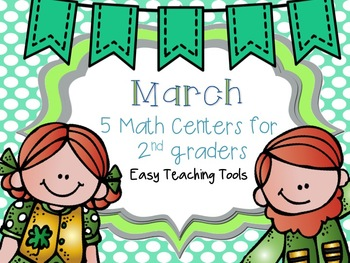# St. Patrick's Day Math Centers {engaging & CCSS aligned}Subjects
Resource Type
Formats Included
PDF (4 MB|38 pages)
Standards
\$3.00
\$3.00

#### Also included in

1. These 2nd grade math centers are perfect for reteaching, independent practice, and centers. They're easy to prep. and something your students will love. Math Centers are also perfect for your early finishers.See how we use 2nd grade math centers here.See how to organize 2nd grade math centers here
\$27.00
\$53.50
Save \$26.50

### Description

I'm in love with math centers as much as reading centers. They're a great review of skills students need to be successful. These math centers will be a wonderful addition to your March calendar!

Includes 5 math centers

-Odd-Even Flip Book(2 versions)

Includes a number version and solving addition and subtraction problems. Students will determine if the number/answer is odd or even

double-digit subtraction with and without regrouping (1, 2, and 3-digit numbers)

doubles

arrays/counting by 2s, 5s, and 10s

3-step problems

money

elapsed time

-Double-digit math game

-Money Review

Students will take an amount card and show the amount in two different ways using fake coins or drawing coins (on the recording sheet provided). You can laminate the recording sheet for multiple uses!

-Graphing(3 versions)

There are 3 different graphs and different recording sheets. Students will interpret data from the graph mats and record the data by creating a bar graph. Students will answer questions about the data.

I hope your students love this activity as much as mine.

Mahalo,

Kristen

Easy Teaching Tools

Get more COMMON CORE MATH RESOURCES in a bundle for a HUGE discount

Winter Math Centers

What Time is It?

Place Value Pals

St. Patrick's Day Math Centers

April Math Centers

Jelly Bean Fraction Game

April Math Centers

Measurement Under Construction

Total Pages
38 pages
Included
Teaching Duration
N/A
Report this Resource to TpT
Reported resources will be reviewed by our team. Report this resource to let us know if this resource violates TpT’s content guidelines.

### Standards

to see state-specific standards (only available in the US).
Determine whether a group of objects (up to 20) has an odd or even number of members, e.g., by pairing objects or counting them by 2s; write an equation to express an even number as a sum of two equal addends.
Fluently add and subtract within 20 using mental strategies. By end of Grade 2, know from memory all sums of two one-digit numbers.
Use addition and subtraction within 100 to solve one- and two-step word problems involving situations of adding to, taking from, putting together, taking apart, and comparing, with unknowns in all positions, e.g., by using drawings and equations with a symbol for the unknown number to represent the problem.
Add and subtract within 1000, using concrete models or drawings and strategies based on place value, properties of operations, and/or the relationship between addition and subtraction; relate the strategy to a written method. Understand that in adding or subtracting three-digit numbers, one adds or subtracts hundreds and hundreds, tens and tens, ones and ones; and sometimes it is necessary to compose or decompose tens or hundreds.
Fluently add and subtract within 100 using strategies based on place value, properties of operations, and/or the relationship between addition and subtraction.